# Quartz.

This page illustrates the conoscopy of quartz for different section thickness. In figure 1, we have a medium section thickness (more or less one millimeter) where isochromes of several orders can be seen. The isogyres are relatively diffuse at the center of the figure. A thicker crystal (a few millimeters thickness), as shown in figure 2, exhibits a much higher number of extinction rings and high order interference colors towards the circumference of the figure. The rings are very well defined in monochromatic light (figure 2b). The quartz crystal  of figure 3 was obtained from a thin section of rock, thickness 30µ. In this figure, the thickness is not high enough to see the first extinction ring so the isochromes are absent from the figure.

Quartz has a positive optical sign as can be seen by the increase of the retardation (blue color) observed in figure 1b along the high index of the wave plate (SW-NE orientation) or the black spots in the quarter wave plate image (figure 1c) appearing in a direction normal to the high index of the accessory plate. The same is true for the thick plate: see for instance the appearance of two black rings along AB in figure 2c. For the thin section, nothing can be seen with a quarter wave plate because the black spots of figure 1c are now at greater distance from the center and are situated out of the field of view. With the wave plate, the blue color along the high index of the plate is the characteristic of a positive crystal optics.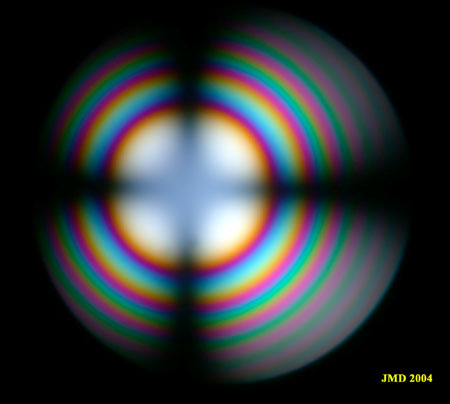Figure 1a. Section of a quartz crystal nearly normal to optic axis.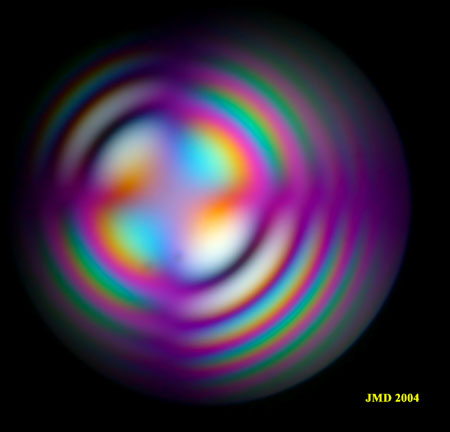Figure 1 b. Addition of a wave plate to figure 1a.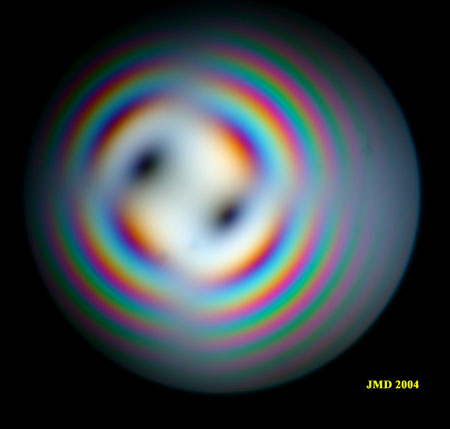Figure 1c. Same section with a quarter wave plate.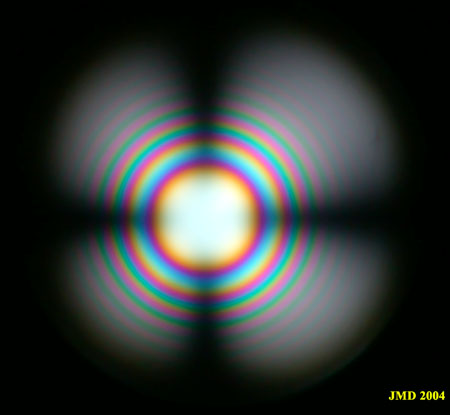Figure 2a. Thick section in a quartz crystal normal to optic axis.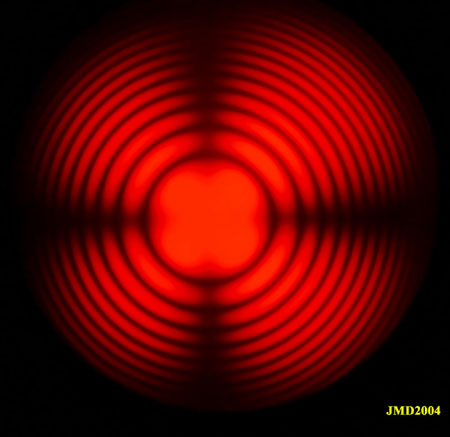Figure 2b. Same section in monochromatic light (628 nm).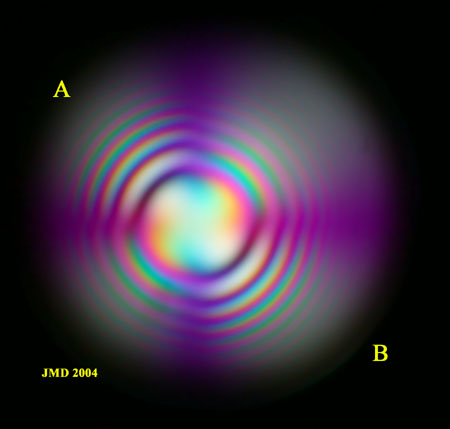Figure 2c. Addition of a wave plate to section 2a.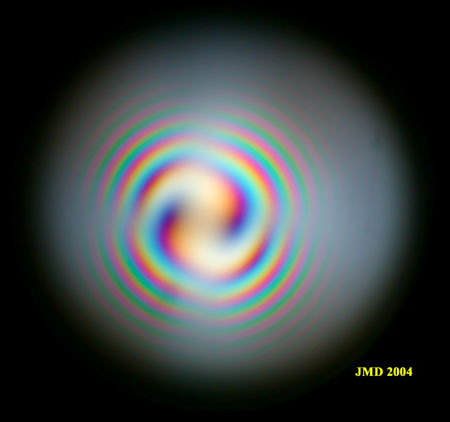Figure 2d. Addition of a quarter wave plate.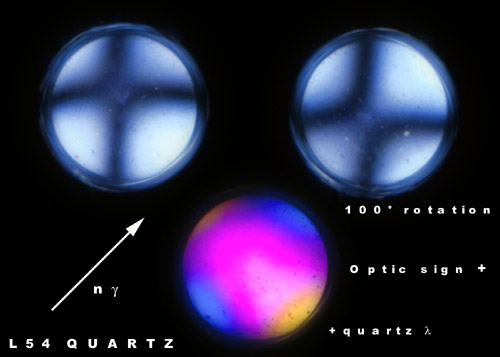Figure 3. Quartz crystal from a plutonic rock thin section (30µ) cut almost perpendicular to the optical axis. The section is so thin that the isochromes are not visible, only the isogyres can be found. During the rotation of the stage, the black cross characteristic of an uniaxial crystal changes to slightly displaced hyperbolae due to anisotropic tensions in the crystal. Note that the rotation angle of the maximum separation of the hyperboles is very different from 45°. The figure at the bottom is produced by the insertion of a first order red plate. The blue color in the direction of the high index of the plate is the diagnostic of a positive optic sign.